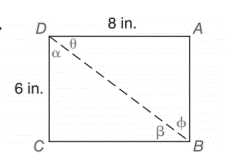Chapter 11.2, Problem 28E### Elementary Geometry for College St...

6th Edition
Daniel C. Alexander + 1 other
ISBN: 9781285195698

#### Solutions

Chapter
Section### Elementary Geometry for College St...

6th Edition
Daniel C. Alexander + 1 other
ISBN: 9781285195698
Textbook Problem
1 views

# In Exercise 23 to 28, use either the sine ratio or the cosine ratio as needed to find the measure of each indicated angle to the nearest degree.To determine

To find:

The measure of each indicated angle of the following triangle,Explanation

Consider the following figure,

General formula for cosine ratio and sin ratio is given below,

In a rectangle opposite sides are equal. That is, AD=BC=8in.andCD=AB=6in.

The Pythagorean theorem is given below,

(BD)2=(CD)2+(BC)2

Substitute the values of AD=BC=8in.andCD=AB=6in. to get the following,

(BD)2=(6)2+(8)2(BD)2=36+64(BD)2=100BD=10in.

Hence 10 in. is the length of leg hypotenuse.

From the given figure the value 10 in. is the hypotenuse value since it is opposite to right angle.

Then 6 in. is the length of the leg adjacent to α and 6 in. is the length of leg opposite to β. Then 8 in. is the length of the leg adjacent to θ and 8 in. is the length of leg opposite to ϕ.

Therefore,

cosα=610cosα=35α=cos1(35)

Using a graphing calculator in degree mode, follow the following key sequence,

cos1(3/5)enter53

So the value of α is given below,

α=53

Therefore,

### Still sussing out bartleby?

Check out a sample textbook solution.

See a sample solution

#### The Solution to Your Study Problems

Bartleby provides explanations to thousands of textbook problems written by our experts, many with advanced degrees!

Get Started

#### Find more solutions based on key concepts